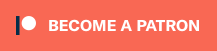# Klipper Extruder Rotation Distance Calculator

Since I started using Klipper more often, and making config files for other printers, I always need to google how to calculate rotation distance for the extruder. To make things easier for me, I thought about having a handy calculator to tell me the exact rotation distance to be used, according to the calibrated E steps.

The calculator available below should make the extruder rotation distance calculation easier.

## How to calculate the Extruder Rotation Distance for Klipper?

To calculate the extruder rotation distance, we need to have the following information:

• Full steps/rotation – determined by the type of stepper motor used.
• Microsteps – determined by the stepper motor driver configuration.
• Steps/mm – determined after calibrating the E-steps of your extruder.

The formula for calculating the extruder rotation distance value to be used in the printer.cfg file is the following:

``rotation_distance = <full_steps_per_rotation> * <microsteps> / <steps_per_mm>``

Let’s take the popular BMG Extruder which has an E step value of 415 when combined with a regular 1.8-degree stepper motor and the stepper driver is set to 16 microsteps. This translates into a rotation distance of 7.710843373493976.

Now, we take this value and add it under the [extruder] section of your printer.cfg file. I recommend using three decimals for the calculated extruder rotation distance. So, the value for the BMG extruder will be 7.710.

``````[extruder]
microsteps: 16
rotation_distance: 7.710
full_steps_per_rotation: 200
``````

Please note that this way of calculating the rotation distance value gives you an approximation of the final value based on the E steps provided. For more accurate calibration of the final rotation_distance for the extruder, use the “measure and trim” method.

There’s no need for me to reiterate what the excellent Klipper documentation also mentions, so if you’re looking for more information, I recommend checking out the official documentation.

## What’s next?

Now that your extruder rotation distance has been calculated and correctly set in the configuration file, I recommend you also fine tune the flow rate.

####Subscribe
Notify of1 Comment
Inline Feedbacks